# C Program to implement HEAP sort Algorithm

In this tutorial you will learn about the C Program to implement HEAP sort Algorithm and its application with practical example.

## C Program to implement HEAP Sort Algorithm

In this tutorial, we will learn to create a C program that will do HEAP sorting using C programming.

## Prerequisites

Before starting with this tutorial we assume that you are best aware of the following C programming topics:

• Operators in C Programming.
• Basic Input and Output function in C Programming.
• Basic C programming.
• For loop in C Programming.
• Creating and Using the user-defined function in C programming.

## What is Heap Sort?

In every programming language, the sorting of data is a very important factor.  In C Language. Many different techniques for sorting are available to work with but in this tutorial, we will focus on the Heap Sort Technique.

This technique is opposite to the selection sort technique. Here, we will select the highest value and put it in last, and create a heap for maximum values. This processing is continued till the smallest value is found.

## Algorithm

Heap Sort

MaxiHeap (arry)

MaxiHeapify(arry,i)

## Output:-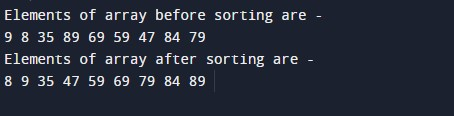In the above program, we have first declared and initialized a set of variables required in the program.

• n = it will hold the size of the array.
• arr[] = it will hold the value of the array.
• x[]= it will hold the elements in an array.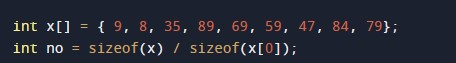Function to print array:-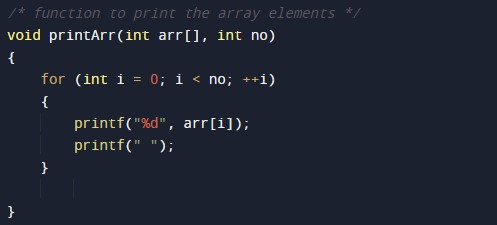Function For Heaping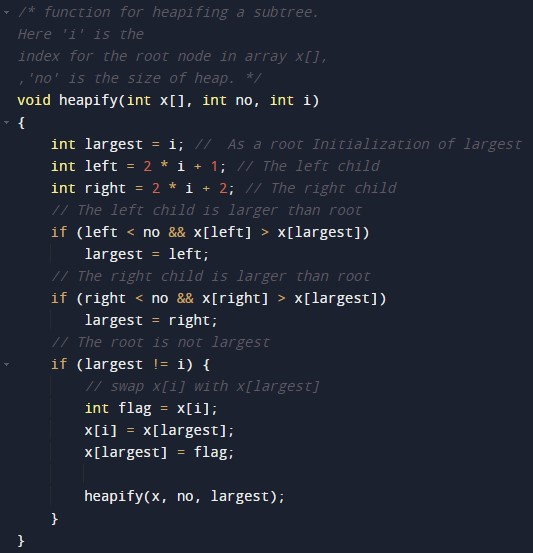HEAP sort functionMain function body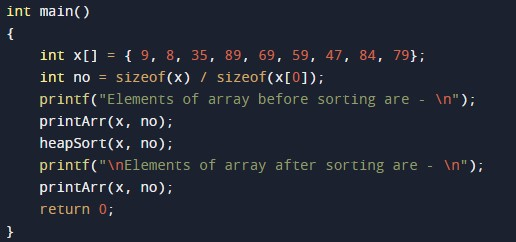In this tutorial we have learn about the C Program to implement HEAP sort Algorithm and its application with practical example. I hope you will like this tutorial.